## Clicker 1

• Use the standard reaction enthalpies given below to determine ΔH°rxn for the following reaction 2 NO(g) + O2(g) → 2 NO2(g) ΔH°rxn = ?
• Given:
• N2(g) + O2(g) → 2 NO(g) ΔH°rxn = +183 kJ
• 1/2 N2(g) + O2(g) → NO2(g) ΔH°rxn = +33 kJ
• A) -150. kJ
• B) -117 kJ
• C) -333 kJ
• D) +115 kJ
• E) +238 kJ

## Standard Conditions and Standard Enthalpy of Formation (ΔHf)

• Audio 0:00:21.144316
• The standard state is the state of a material at a defined set of conditions.
• Pure gas at exactly 1 atm pressure
• Pure solid or liquid in its most stable form at exactly 1 atm pressure and temperature of interest
• Usually 25 °C
• Substance in a solution with concentration 1 M
• The standard enthalpy change, ΔH°, is the enthalpy change when all reactants and products are in their standard states.
• Audio 0:02:59.978786
• The standard enthalpy of formation, ΔH°f, is the enthalpy change for the reaction forming 1 mole of a pure compound from its constituent elements.
• The elements must be in their standard states.
• The ΔHf° for a pure element in its standard state = 0 kJ/mol.

## Table of Standard Enthalpies (ΔHf)

• Audio 0:05:22.505566
•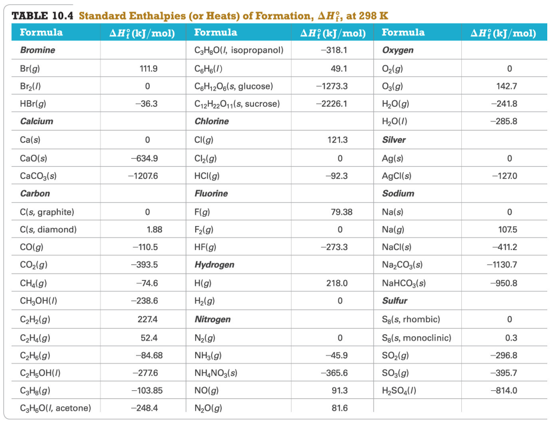## Practice Problem: Standard Enthalpies of Formation (ΔHf)

• Audio 0:08:11.763970
• Write the appropriate equations for the heats of formation of MgCO3(s) and C6H12O6(s)

## Clicker 2

• The standard enthalpy of formation (ΔHo f) for potassium chloride is the enthalpy change for the reaction:
• A) K(g) + ½Cl2(g) è KCl(g)
• B) K+(g) + Cl- (g) è KCl(s)
• C) 2K(s) + Cl2(g) è 2KCl(s)
• D) K(s) + ½Cl2(g) è KCl(s)
• E) K+(g) + Cl- (g) è KCl(g)

## Calculating Standard Enthalpy Change for a Reaction

• Audio 0:12:36.053007
• Any reaction can be written as the sum of formation reactions (or the reverse of formation reactions) for the reactants and products.
• The ΔH° for the reaction is then the sum of the ΔHf° for the component reactions. ΔH° reaction = ∑nΔHf °(products) − ∑nΔHf °(reactants) ∑ means sum. n is the stoichiometric coefficient of the reaction.

## CH4(g) + 2 O2(g) → CO2(g) + 2H2O(g)

• C(s, graphite) + 2 H2(g) → CH4(g) ΔHf°= − 74.6 kJ/mol CH4
• C(s, graphite) + O2(g) → CO2(g) ΔHf°= −393.5 kJ/mol CO2
• H2(g) + ½ O2(g) → H2O(g) ΔHf° = −241.8 kJ/mol H2O

## CH4(g) + 2 O2(g) → CO2(g) + 2H2O(g)

• Audio 0:17:54.502946
•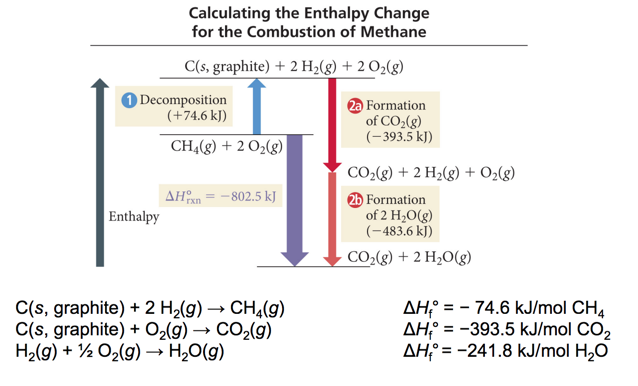•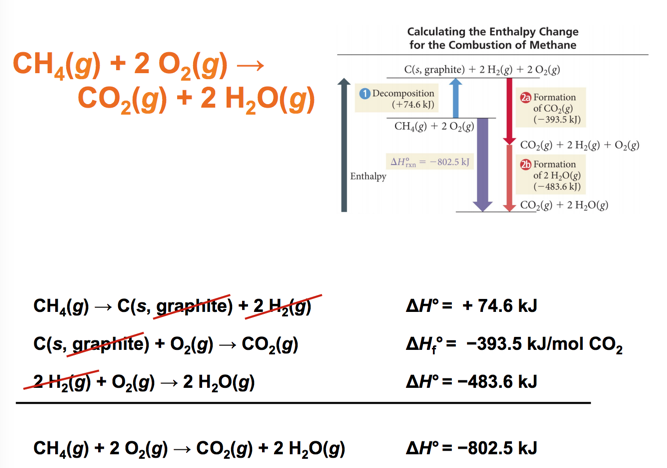## Practice Problem: Standard Enthalpies of Formation (ΔHf )

• Audio 0:18:40.315050
• What is the change in enthalpy for the reaction 4NH3(g) + 5O2(g) è 4NO(g) + 6H2O(g)

## Clicker 3

• Audio 0:21:26.032462
• Use the ΔH°f information provided to calculate ΔH°rxn for the following SO2Cl2(g) + 2 H2O(l) → 2 HCl(g) + H2SO4(l) ΔH°rxn = ?
• ΔH° f (kJ/mol)
• SO2Cl2(g) -364
• H2O(l) -286
• HCl(g) -92
• H2SO4(l) -814
• A) -256 kJ
• B) +161 kJ
• C) -62 kJ
• D) +800. kJ
• E) -422 kJ

## Ionic Bonding and the Crystal Lattice

• Audio 0:26:20.219643
• The extra energy that is released comes from the formation of a structure in which every cation is surrounded by anions, and vice versa.
• This structure is called a crystal lattice.
• The crystal lattice is held together by the electrostatic attraction of the cations for all the surrounding anions.
• The crystal lattice maximizes the attractions between cations and anions, leading to the most stable arrangement.

## Lattice Energy

• The extra stability that accompanies the formation of the crystal lattice is measured as the lattice energy.
• The lattice energy is the energy released when the solid crystal forms from separate ions in the gas state.
• Always exothermic
• Hard to measure directly, but can be calculated from knowledge of other processes
• Lattice energy depends directly on the size of charges and inversely on distance between ions.

## Determining Lattice Energy: The Born–Haber Cycle

• The Born–Haber cycle is a hypothetical series of reactions that represents the formation of an ionic compound from its constituent elements.
• The reactions are chosen so that the change in enthalpy of each reaction is known except for the last one, which is the lattice energy.

## Born–Haber Cycle and Hess’s Law

•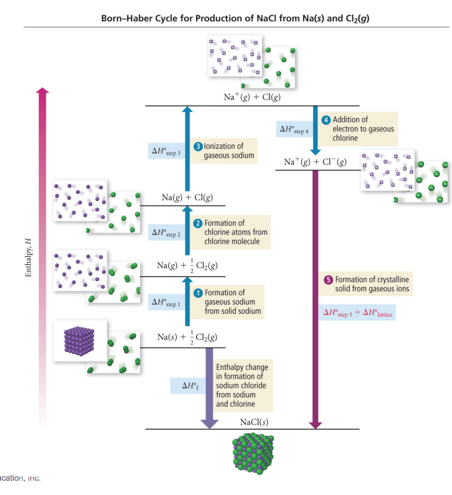• Use Hess’s law to add up enthalpy changes of other reactions to determine the lattice energy.
•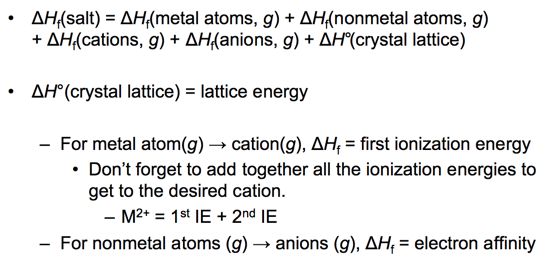• Audio 0:31:13.639819
• The force of attraction between charged particles is inversely proportional to the distance between them.
• Larger ions mean the center of positive charge (nucleus of the cation) is farther away from the negative charge (electrons of the anion).
• Larger ion = weaker attraction
• Weaker attraction = smaller lattice energy

## Lattice Energy versus Ion Size

• Audio 0:31:57.501608
• The force of attraction between oppositely charged particles is directly proportional to the product of the charges.
• Larger charge means the ions are more strongly attracted.
• Larger charge = stronger attraction
• Stronger attraction = larger lattice energy
•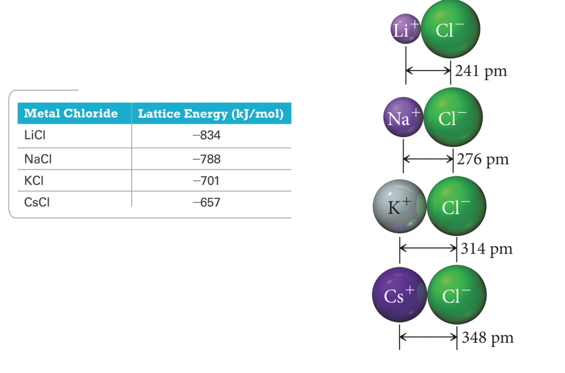• The force of attraction between oppositely charged particles is directly proportional to the product of the charges.
• Larger charge means the ions are more strongly attracted.
• Larger charge = stronger attraction
• Stronger attraction = larger lattice energy
• Of the two factors, ion charge is generally more important.
•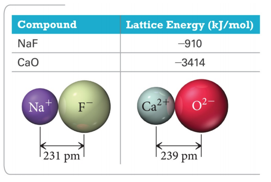# Ch 11

• What is a Gas?

# Vocab

Term Definition
bond energy amount of energy it takes to break one mole of a bond in a compound
standard state state of a material at a defined set of conditions
standard enthalpy change (ΔH°) the enthalpy change when all reactants and products are in their standard states
standard enthalpy of formation (ΔH°f) the enthalpy change for the reaction forming 1 mole of a pure compound from its constituent elements
Born–Haber cycle a hypothetical series of reactions that represents the formation of an ionic compound from its constituent elements